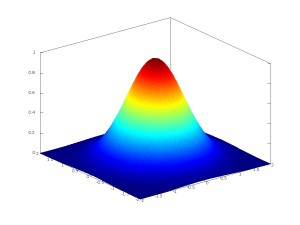Few days ago Arnaud Guillin uploaded the joint paper arXiv:1104.2198 with Patrick Cattiaux entitled Central limit theorems for additive functionals of ergodic Markov diffusions processes. In this paper, we revisit functional central limit theorems for additive functionals of ergodic Markov diffusion processes. Translated in the language of partial differential equations of evolution, they appear as diffusion limits in the asymptotic analysis of Fokker-Planck type equations. We focus on the square integrable framework, and we provide tractable conditions on the infinitesimal generator, including degenerate or anomalously slow diffusions. We take advantage on recent developments in the study of the trend to the equilibrium of ergodic diffusions via functional inequalities and Lyapunov criteria. We discuss examples and formulate open problems.

Let ${{(X_t)}_{t\geq0}}$ be a continuous time strong Markov process with state space ${\mathbb{R}^d}$, non explosive, irreducible, positive recurrent, with unique invariant probability measure ${\mu}$. For every ${f\in\mathbb{L}^1(\mu)}$, if almost surely (a.s.) the function ${s\in\mathbb{R}_+\mapsto f(X_s)}$ is locally Lebesgue integrable, then

$\frac{S_t}{t} \underset{t\rightarrow\infty}{\overset{\text{a.s.}}{\longrightarrow}} \int\!f\,d\mu \quad\text{where}\quad S_t:=\int_0^t\!f(X_s)\,ds. \ \ \ \ \ (1)$

If ${X_0\sim\mu}$ then by the Fubini theorem (1) holds for all ${f\in\mathbb{L}^1(\mu)}$ and the convergence holds additionally in ${\mathbb{L}^1}$ thanks to the dominated convergence theorem. The statement (1) which relates an average in time with an average in space is an instance of the ergodic phenomenon. It can be seen as a strong law of large numbers for the additive functional ${{(S_t)}_{t\geq0}}$ of the Markov process ${{(X_t)}_{t\geq0}}$. The asymptotic fluctuations are described by a central limit theorem which is the subject of this work. Let us assume that ${X_0\sim\mu}$ and ${f\in\mathbb{L}^2(\mu)}$ with ${\int\!f\,d\mu=0}$ and ${f\neq0}$. Then for all ${t\geq0}$ we have ${S_t\in\mathbb{L}^2(\mu)\subset\mathbb{L}^1(\mu)}$ and ${\mathbb{E}(S_t)=0}$. We say that ${{(S_t)}_{t\geq0}}$ satisfies to a central limit theorem (CLT) when

$\frac{S_t}{s_t} \overset{\text{law}}{\underset{t\rightarrow\infty}{\longrightarrow}} \mathcal{N}(0,1) \ \ \ \ \ (2)$

for a deterministic positive function ${t\mapsto s_t}$ which may depend on ${f}$. Here ${\mathcal{N}(0,1)}$ stands for the standard Gaussian law on ${\mathbb{R}}$ with mean ${0}$ and variance ${1}$. By analogy with the CLT for i.i.d. sequences one may expect that ${s_t^2=\mathrm{Var}(S_t)}$ and that this variance is of order ${t}$ as ${t\rightarrow\infty}$. A standard strategy for proving (2) consists in representing ${{(S_t)}_{t\geq0}}$ as a sum of an ${\mathbb{L}^2}$-martingale plus a remainder term which vanishes in the limit, reducing the proof to a central limit theorem for martingales. This strategy is particularly simple under mild assumptions, see for instance the book of Jacod and Shiryaev (VII.3 page 486). Namely, if ${L}$ is the infinitesimal generator of ${{(X_t)}_{t\geq0}}$ with domain ${\mathbb{D}(L)\subset\mathbb{L}^2(\mu)}$ and if ${g\in\mathbb{D}(L)}$ then ${{(M_t)}_{t\geq0}}$ defined by

$M_t:=g(X_t)-g(X_0)-\int_0^t\!(Lg)(X_s)\,ds$

is a local ${\mathbb{L}^2}$ martingale. Now if ${g^2\in\mathbb{D}(L)}$ and

$\Gamma(g):=L(g^2)-2gLg\in\mathbb{L}^1(\mu)$

then

$\left<M\right>_t=\int_0^t\!\Gamma(g)(X_s)\,ds.$

The law of large numbers (1) yields

$\lim_{t\rightarrow\infty}\frac{1}{t}\left<M\right>_t=\int\!\Gamma(g)\,d\mu.$

As a consequence, for a prescribed ${f}$, if the Poisson equation

$Lg=f$

admits a mild enough solution ${g}$ then

$\frac{M_t}{s_t}=\frac{g(X_t)-g(X_0)}{s_t}-\frac{S_t}{s_t}.$

This suggests to deduce (2) from a CLT for martingales (survey paper by Ward). We begin by revisiting this strategy. Beyond (2), we say that ${{(S_t)}_{t\geq0}}$ satisfies to a Functional Central Limit Theorem (FCLT) or Invariance Principle when for every finite sequence ${0<t_1\leq\cdots\leq t_n<\infty}$,

$\left(\frac{S_{t_1/\varepsilon}}{s_{t_1/\varepsilon}}, \ldots,\frac{S_{t_n/\varepsilon}}{s_{t_n/\varepsilon}}\right) \overset{\text{law}}{\underset{\varepsilon\rightarrow0}{\longrightarrow}} \mathcal{L}\left(\left(B_{t_1},\ldots,B_{t_n}\right)\right) \ \ \ \ \ (3)$

where ${{(B_t)}_{t\geq0}}$ is a standard Brownian Motion on ${\mathbb{R}}$. Taking ${n=1}$ gives (2). To capture multitime correlations, one may upgrade the convergence in law in (3) to an ${\mathbb{L}^2}$ convergence. The statement (3) means that as ${\varepsilon\rightarrow0}$, the rescaled process ${{(S_{t/\varepsilon}/s_{t/\varepsilon})}_{t\geq0}}$ converges in law to a Brownian Motion, for the topology of finite dimensional marginal laws. At the level of Chapman-Kolmogorov-Fokker-Planck equations, (3) is a diffusion limit for a weak topology.

We focus on the case where ${{(X_t)}_{t\geq0}}$ is a Markov diffusion process on ${E=\mathbb{R}^d}$, and we seek for conditions on ${f}$ and on the infinitesimal generator in order to get (2) or even (3). We revisit the renowned result of Kipnis and Varadhan, and provide an alternative approach which is not based on the resolvent. Our results cover fully degenerate situations such as kinetic models. More generally, we believe that a whole category of diffusion limits which appear in the asymptotic analysis of evolution partial differential equations of Fokker-Planck type enters indeed the framework of the central limit theorems we shall discuss. We also explain how the behavior out of equilibrium (i.e. ${X_0\not\sim\mu}$) may be recovered from the behavior at equilibrium (i.e. ${X_0\sim\mu}$) by using propagation of chaos (decorrelation), for instance via Lyapunov criteria ensuring a quick convergence in law of ${X_t}$ to ${\mu}$ as ${t\rightarrow\infty}$. Note that since we focus on an ${\mathbb{L}^2}$ framework, the natural normalization is the square root of the variance and we can only expect Gaussian fluctuations. We believe however that stable limits that are not Gaussian, also known as “anomalous diffusion limits”, can be studied using similar tools, see for instance the forthcoming book Fluctuations in Markov Processes by Landim, Olla, and Komorowski.

This site uses Akismet to reduce spam. Learn how your comment data is processed.

Syntax · Style · .